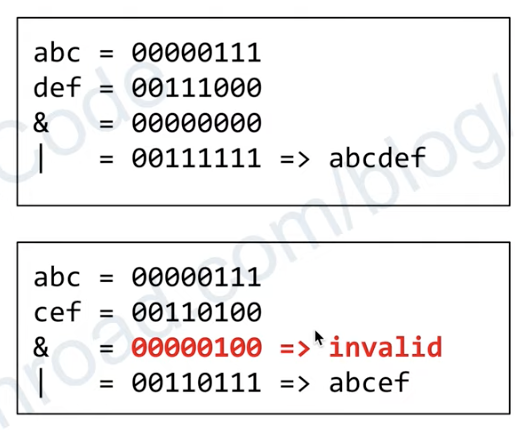# 1239.Maximum Length of a Concatenated String with Unique Characters

September 22, 2021

### Description:

Given an array of strings arr. String s is a concatenation of a sub-sequence of arr which have unique characters.

Return the maximum possible length of s.

#### Sample Input:

Constraints:

• 1 <= arr.length <= 16
• 1 <= arr[i].length <= 26
• arr[i] contains only lower case English letters.

#### Note:

• A subsequence should preserve the order.

• For example, arr = [“un”,”iq”,”ue”], “iqun” is not a valid answer.
• Corollary:
• However, we only want the length of the string, so the order of the strings are trivial.
• The constraints has implied us that the algorithm could be exponential.

• if arr[i] itself contains duplicate characters, it’s invalid.

### BruteForce:

Traverse all the string pair, find if the concantenated string has duplicate char.

Time Complexity $O(2^n)$ : each string can be cancatenated or not.

### Technique:

Since the arr[i].length<=26<32, we can use a 32bit int to do the char count

(How many times each character present in a string instead of map)

Time Complexity $O(2^n)$ : each string can be cancatenated or not.

Space Complexity $O(n)$ : using DFS recursion, the space complexity equals to the depth of recursion.

#### Sample:Referenced from Hua Hua@Youtube